# ISEE Lower Level Quantitative : How to compare fractions

## Example Questions

### Example Question #2764 : Isee Lower Level (Grades 5 6) Quantitative Reasoning

Select the symbol to correctly fill in the blank below.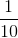__________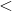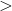Explanation:

To compare fractions, we need to first make common denominators.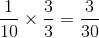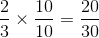Now that we have common denominators, we can compare numerators. The fraction with the bigger numerator has the greater value.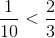### Example Question #2765 : Isee Lower Level (Grades 5 6) Quantitative Reasoning

Select the symbol to correctly fill in the blank below.__________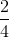Explanation:

To compare fractions, we need to first make common denominators.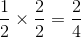Now that we have common denominators, we can compare numerators. The fraction with the bigger numerator has the greater value.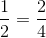### Example Question #2766 : Isee Lower Level (Grades 5 6) Quantitative Reasoning

Select the symbol to correctly fill in the blank below.__________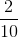Explanation:

To compare fractions, we need to first make common denominators.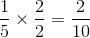Now that we have common denominators, we can compare numerators. The fraction with the bigger numerator has the greater value.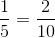### Example Question #2767 : Isee Lower Level (Grades 5 6) Quantitative Reasoning

Select the symbol to correctly fill in the blank below.__________Explanation:

To compare fractions, we need to first make common denominators.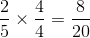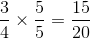Now that we have common denominators, we can compare numerators. The fraction with the bigger numerator has the greater value.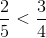### Example Question #2768 : Isee Lower Level (Grades 5 6) Quantitative Reasoning

Select the symbol to correctly fill in the blank below.__________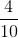Explanation:

To compare fractions, we need to first make common denominators.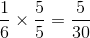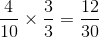Now that we have common denominators, we can compare numerators. The fraction with the bigger numerator has the greater value.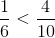### Example Question #2769 : Isee Lower Level (Grades 5 6) Quantitative Reasoning

Select the symbol to correctly fill in the blank below.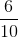__________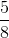Explanation:

To compare fractions, we need to first make common denominators.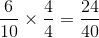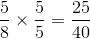Now that we have common denominators, we can compare numerators. The fraction with the bigger numerator has the greater value.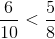### Example Question #2770 : Isee Lower Level (Grades 5 6) Quantitative Reasoning

Select the symbol to correctly fill in the blank below.__________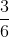Explanation:

To compare fractions, we need to first make common denominators.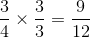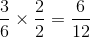Now that we have common denominators, we can compare numerators. The fraction with the bigger numerator has the greater value.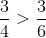### Example Question #2771 : Isee Lower Level (Grades 5 6) Quantitative Reasoning

Select the symbol to correctly fill in the blank below.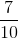__________Explanation:

To compare fractions, we need to first make common denominators.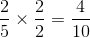Now that we have common denominators, we can compare numerators. The fraction with the bigger numerator has the greater value.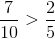### Example Question #2772 : Isee Lower Level (Grades 5 6) Quantitative Reasoning

Select the symbol to correctly fill in the blank below.__________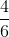Explanation:

To compare fractions, we need to first make common denominators.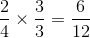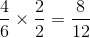Now that we have common denominators, we can compare numerators. The fraction with the bigger numerator has the greater value.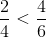### Example Question #2773 : Isee Lower Level (Grades 5 6) Quantitative Reasoning

Select the symbol to correctly fill in the blank below.__________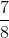Explanation:

To compare fractions, we need to first make common denominators.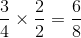Now that we have common denominators, we can compare numerators. The fraction with the bigger numerator has the greater value.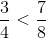### All ISEE Lower Level Quantitative Resources